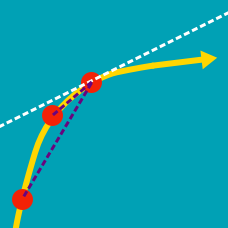Calculus

# Graphical Interpretation of Derivatives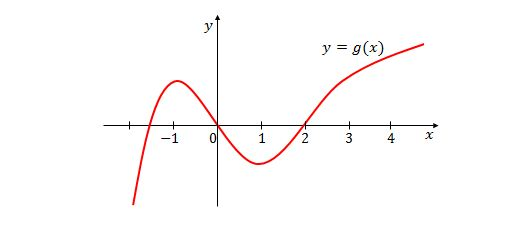The above graph represents the function $y=g(x).$ Judging the graph as well as you can by eye, what can we say about the derivatives of $g(x)$ at $x=-2,0,2?$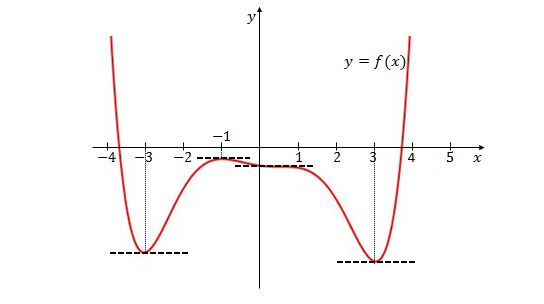If the graph of $y=f(x)$ is as shown above, on which of the following intervals is the slope of the tangent line always positive?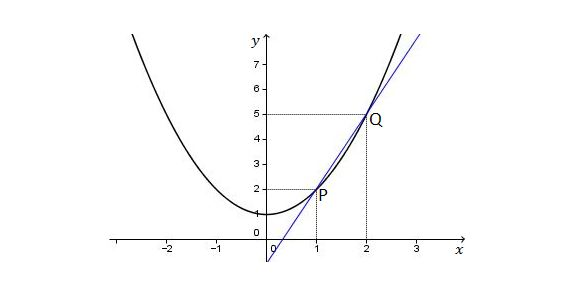Which of the following is a possible value for the slope of the tangent line to the above curve at point $P=(1, 2) ?$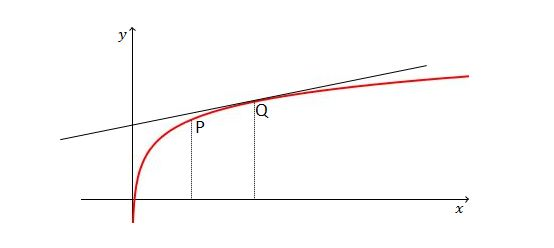If the equation of the tangent line at point $Q$ is $y=0.924x+6,$ which of the following is a possible value for the slope of the tangent line at $P ?$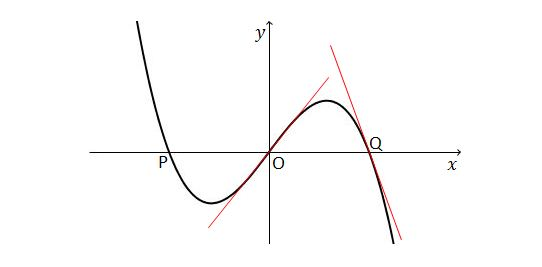The above diagram is the graph of a function $f$ satisfying $f(-x) = -f(x)$. If the slope of the tangent line at point $O$ is $1.542$ and the slope of the tangent line at point $Q$ is $-1.316,$ what is the slope of the tangent line at point $P ?$

×